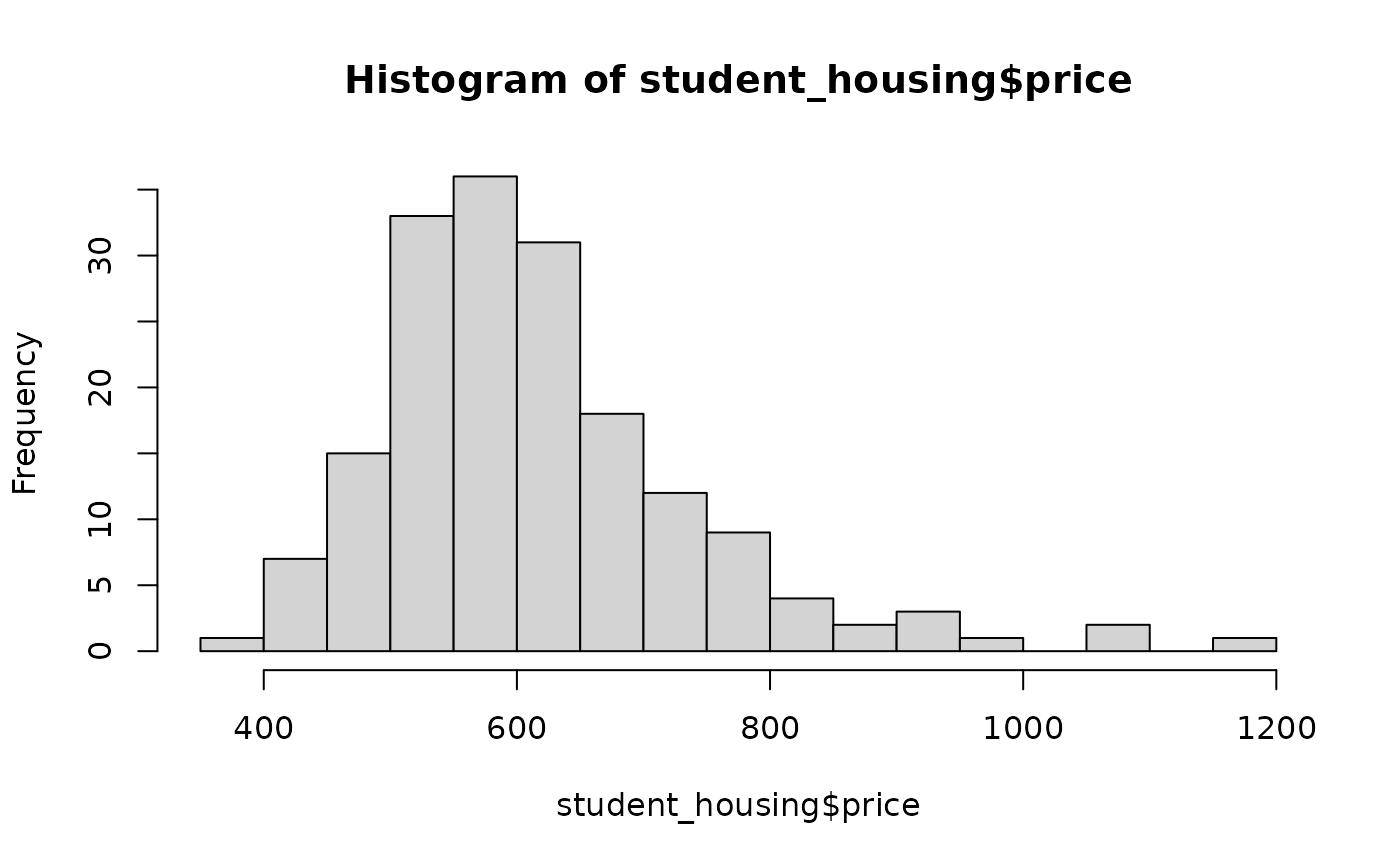These are simulated data and intended to represent housing prices of students at a college.

## Usage

student_housing

## Format

A data frame with 175 observations on the following variable.

price

Monthly housing price, simulated.

## Examples


set.seed(5)
generate_student_housing <- data.frame(
price = round(rnorm(175, 515, 65) + exp(rnorm(175, 4.2, 1)))
)
hist(student_housing$price, 20)t.test(student_housing$price)
#>
#> 	One Sample t-test
#>
#> data:  student_housing$price #> t = 63.433, df = 174, p-value < 0.00000000000000022 #> alternative hypothesis: true mean is not equal to 0 #> 95 percent confidence interval: #> 597.7138 636.1034 #> sample estimates: #> mean of x #> 616.9086 #> mean(student_housing$price)
#>  616.9086
sd(student_housing\$price)
#>  128.6541
identical(student_housing, generate_student_housing)
#>  FALSE